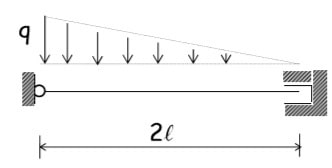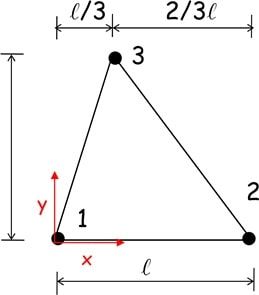# Supplementary exercise

## Supplementary exercise 1E,A,I

For the represented structure evaluate:

• the response in terms of internal forces and displacement distribution
• the value and the position along the beam axis of the maximum displacement

in the hypothesis of a slender beam discretized by a single element.

Compare the numerical results to the corresponding analytical solution.

Draw the graphs of the internal forces and sketch the deformed configuration of the beam axis for both the exact and the approximated numerical solution.

## Supplementary exercise 2Evaluate the nodal forces equivalent to the self-weight assuming that gravity is parallel to y, specific weight (weight per unit volume) is γ and element thickness varies along y according to the rule: h(y)=h0(1+y/ ).